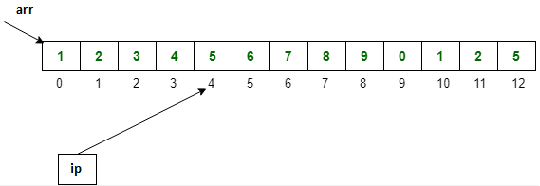# GATE Solved Paper 2017-19 - GATE 2019

• A

1• B

2• C

3• D

4• Option : B
• Explanation :
According to chinese remainder theorem:
= 351 mod 5
= (33)17 mod 5
= (27)17 mod 5
= {(22)8*21} mod 5
= {(4)8*(2)} mod 5
= {(-1)8*(2)} mod 5
= {(1*2)} mod 5
= {(2)} mod 5
= 2

• A

0.5029• B

0.538• C

0.461• D

0.248• Option : A
• Explanation :
The 4-bit binary representation of numbers (1, 2, 3, 4………13):
0 - 0000
1 - 0001
2 - 0010
3 - 0011
4 - 0100
5 - 0101
6 - 0110
7 - 0111
8 - 1000
9 - 1001
10 - 1010
11 - 1011
12 - 1100
13 - 1101
There 6 numbers which start with MSB as 1, and 7 numbers which start with MSB as 0.
Therefore, probability that their 4-bit binary representations have the same most significant bit is,
= P(MSB is 0) + P(MSB is 1)
= (7×7)/(13×13) + (6×6)/(13×13)
= (49+36)/169
= 85/169
= 0.5029
Option (A) is correct.

 P1 P2 P3 : : : : : : D = D + 20 D = D – 50 D = D + 10 : : : : : :
The processes are executed on a uniprocessor system running a time-shared operating system. If the minimum and maximum possible values of D after the three processes have completed execution are X and Y respectively, then the value of Y – X is _____________.

Note – Numerical Type question

• A

80• B

130• C

50• D

None of these• Option : A
• Explanation :
Minimum value (X) of D will possible when,

P2 reads D=100, preempted.
P1 executes D=D+20, D=120.
P3 executes D=D+10, D=130.
Now, P2 has D=100, executes, D = D-50 = 100-50 = 50. P2 writes D=50 final value.
So, minimum value (X) of D is 50.
Maximum value (Y) of D will possible when,

P1 reads D=100, preempted.
P2 reads D=100, executes, D = D-50 = 100-50 = 50.
Now, P1 executes, D = D+20 = 100+20 = 120.
And now, P3 reads D=120, executes D=D+10, D=130. P3 writes D=130 final value.
So, maximum value (Y) of D is 130.

Therefore,
= Y - X
= 130 - 50
= 80
So, option (A) is correct.

int main(){
int arr[]={1,2,3,4,5,6,7,8,9,0,1,2,5},
*ip=arr+4;
printf(“%d\n”, ip);
return 0;
}
The number that will be displayed on execution of the program is ___________ .

Note – Numerical Type question

• A

6• B

5• C

4• D

segmentation error• Option : A
• Explanation :Initially ip pointer is pointing at (arr+4) or skipping starting first 4 position.Now in the printf system call, 1 more position is to skip, So it will point to (arr+5) or skip 5 position from starting:
Hence, printf will print value at 6th position, i.e., 6 will printed.
So, option (A) is correct.

Note – Numerical Type question

• A

29• B

19• C

39• D

09• Option : A
• Explanation :
According to largest Sum Contiguous subarray is from index 2 to 11,
Max (S(i, j))
= S(2, 11)
= 6 + 3 + (-1) + (-2) + 13 + 4 + (-9) + (-1) + 4 + 12
= 29
So, answer is 29.
Related Quiz.
GATE 2019